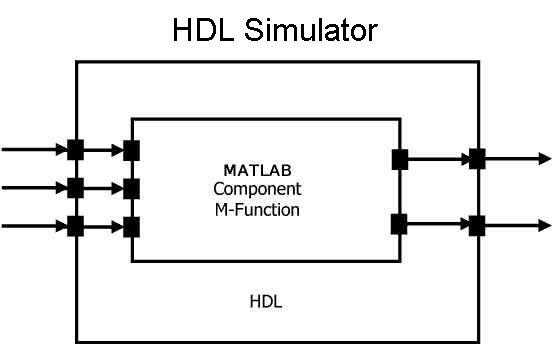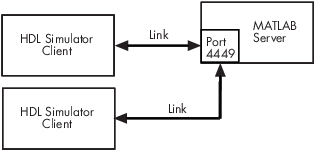## Create a MATLAB Component Function

The HDL Verifier™ software provides a means for visualizing HDL components within the MATLAB® environment. You do so by coding an HDL model and a MATLAB function that can share data with the HDL model. This chapter discusses the programming, interfacing, and scheduling conventions for MATLAB component functions that communicate with the HDL simulator.

MATLAB component functions simulate the behavior of components in the HDL model. A stub module (providing port definitions only) in the HDL model passes its input signals to the MATLAB component function. The MATLAB component processes this data and returns the results to the outputs of the stub module. A MATLAB component typically provides some functionality (such as a filter) that is not yet implemented in the HDL code.

The following figure shows how an HDL simulator wraps around a MATLAB component function and how MATLAB communicates with the HDL simulator during a component simulation session.When linked with MATLAB, the HDL simulator functions as the client, with MATLAB as the server. The following figure shows a multiple-client scenario connecting to the server at TCP/IP socket port 4449.The MATLAB server can service multiple simultaneous HDL simulator sessions and HDL modules. However, you should follow recommended guidelines to help the server track the I/O associated with each module and session. The MATLAB server, which you start with the supplied MATLAB function `hdldaemon`, waits for connection requests from instances of the HDL simulator running on the same or different computers. When the server receives a request, it executes the specified MATLAB function you have coded to perform tasks on behalf of a module in your HDL design. Parameters that you specify when you start the server indicate whether the server establishes shared memory or TCP/IP socket communication links.

Refer to Cosimulation Configurations for valid machine configurations.

### Note

The programming, interfacing, and scheduling conventions for test bench functions and component functions are virtually identical. For the most part, the same procedures apply to both types of functions.

Follow these workflow steps to create a MATLAB component function for cosimulation with the HDL simulator.

1. Create HDL module. Compile, elaborate, and simulate model in HDL simulator . See Write HDL Modules for MATLAB Visualization.

2. Create component MATLAB function. See Write a Component Function.

3. Place component function on MATLAB search path. See Place Component Function on MATLAB Search Path.

4. Bind HDL instance with component function using `matlabcp`. See Bind Component Function Calls With matlabcp.

5. Add scheduling options. See Schedule Options for a Component Session.

6. Set breakpoints for interactive HDL debug (optional).

7. Run cosimulation from HDL simulator. See Run MATLAB-HDL Cosimulation.

### Write HDL Modules for MATLAB Visualization

#### Coding HDL Modules for Visualization with MATLAB

The most basic element of communication in the HDL Verifier interface is the HDL module. The interface passes all data between the HDL simulator and MATLAB as port data. The HDL Verifier software works with any existing HDL module. However, when you code an HDL module that is targeted for MATLAB verification, you should consider its name, the types of data to be shared between the two environments, and the direction modes. The sections within this chapter cover these topics.

The process for coding HDL modules for MATLAB visualization is as follows:

#### Choose HDL Module Name for Use with MATLAB Component Function

Although not required, when naming the HDL module, consider choosing a name that also can be used as a MATLAB function name. (Generally, naming rules for VHDL® or Verilog® and MATLAB are compatible.) By default, HDL Verifier software assumes that an HDL module and its simulation function share the same name. See Bind Test Bench Function Calls With matlabtb.

For details on MATLAB function-naming guidelines, see “MATLAB Programming Tips” on files and file names in the MATLAB documentation.

#### Specify Port Direction Modes in HDL Module for Use with Component Functions

In your module statement, you must specify each port with a direction mode (input, output, or bidirectional). The following table defines these three modes.

Use VHDL Mode...Use Verilog Mode...For Ports That...
`IN``input`Represent signals that can be driven by a MATLAB function
`OUT``output`Represent signal values that are passed to a MATLAB function
`INOUT``inout`Represent bidirectional signals that can be driven by or pass values to a MATLAB function

#### Specify Port Data Types in HDL Modules for Use with Component Functions

Port Data Types for VHDL Entities.  In your entity statement, you must define each port that you plan to test with MATLAB with a VHDL data type that is supported by the HDL Verifier software. The interface can convert scalar and array data of the following VHDL types to comparable MATLAB types:

• `STD_LOGIC`, `STD_ULOGIC`, `BIT`, `STD_LOGIC_VECTOR`, `STD_ULOGIC_VECTOR`, and `BIT_VECTOR`

• `INTEGER` and `NATURAL`

• `REAL`

• `TIME`

• Enumerated types, including user-defined enumerated types and `CHARACTER`

The interface also supports all subtypes and arrays of the preceding types.

### Note

The HDL Verifier software does not support VHDL extended identifiers for the following components:

• Port and signal names used in cosimulation

• Enum literals when used as array indices of port and signal names used in cosimulation

However, the software does support basic identifiers for VHDL.

Port Data Types for Verilog Modules.  In your module definition, you must define each port that you plan to test with MATLAB with a Verilog port data type that is supported by the HDL Verifier software. The interface can convert data of the following Verilog port types to comparable MATLAB types:

• reg

• integer

• wire

### Note

HDL Verifier software does not support Verilog escaped identifiers for port and signal names used in cosimulation. However, it does support simple identifiers for Verilog.

#### Compile and Elaborate HDL Design for Use with Component Functions

After you create or edit your HDL source files, use the HDL simulator compiler to compile and debug the code.

For more examples, see the HDL Verifier tutorials and demos. For details on using the HDL compiler, see the simulator documentation.

### Write a Component Function

#### Overview to Coding an HDL Verifier Component Function

Coding a MATLAB function that is to visualize an HDL module or component requires that you follow specific coding conventions. You must also understand the data type conversions that occur, and program data type conversions for operating on data and returning data to the HDL simulator.

To code a MATLAB function that is to verify an HDL module or component, perform the following steps:

1. Learn the syntax for a MATLAB HDL Verifier component function. See Syntax of a Component Function.

2. Understand how HDL Verifier software converts data from the HDL simulator for use in the MATLAB environment. See Supported Data Types.

3. Choose a name for the MATLAB component function. See Invoke MATLAB Component Function Command matlabcp.

4. Define expected parameters in the component function definition line. See MATLAB Function Syntax and Function Argument Definitions.

5. Determine the types of port data being passed into the function. See MATLAB Function Syntax and Function Argument Definitions.

6. Extract and, if applicable to the simulation, apply information received in the `portinfo` structure. See Gaining Access to and Applying Port Information.

7. Convert data for manipulation in the MATLAB environment, as applicable. See Converting HDL Data to Send to MATLAB.

8. Convert data that needs to be returned to the HDL simulator. See Converting Data for Return to the HDL Simulator.

For more tips, see Test Bench and Component Function Writing.

#### Syntax of a Component Function

The syntax of a MATLAB component function is

```function [iport, tnext] = MyFunctionName(oport, tnow, portinfo) ```

The input/output arguments, `iport` and `oport`, for a MATLAB component function are the reverse of the port arguments for a MATLAB test bench function. That is, the MATLAB component function returns signal data to the outputs and receives data from the inputs of the associated HDL module.

Initialize the function outputs to empty values at the beginning of the function as in the following example:

```tnext = []; oport = struct(); ```

See MATLAB Function Syntax and Function Argument Definitions for an explanation of each of the function arguments. For more information on using `tnext` and `tnow` for simulation scheduling with `matlabcp`, see Schedule Component Functions Using the tnext Parameter.

## Related Topics

##### SupportGet trial now